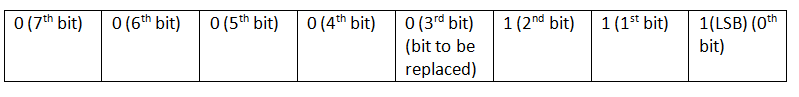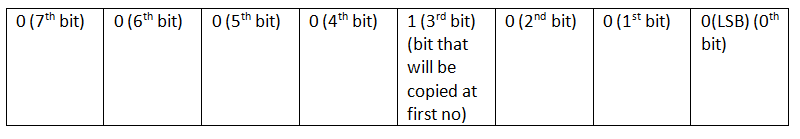# C program to replace bit in an integer at a specified position from another integer

In this article, we are going to learn how to replace a bit of a number at a specified position from another number?
Submitted by Radib Kar, on December 30, 2018

Problem statement: Write a C program to replace specified bit of a number from another number.

Solution: We can use bitwise operator here to solve the problem.

In this solution we have considered 8 bits representation which can be extended up to 32 bits using the same idea.

```    Let first no whose bit is to be replaced is:
7 //0000 0111

Second no from whom bit is to be replaced
8 //0000 1000

Specified position: 3 (0-indxed)
```

First no: 7Second no: 8Algorithm:

```IF specified bit at second no is 1
1)  Form a bit mask (0, d)
0 - for all position except the bit specified
d - 1 at specified position(dth)
2)  Do bitwise OR between the first no and the mask
ELSE //specified bit at second no 0
1)  Form a bit mask (1, d)
1 - For all position except the bit specified
d - 0 at specified position (dth)
2)  Do bitwise AND between the first no & the mask.
```

```Let the specified position d.
Declare temp as (second>>d) & 1
Right shift second number d times to get the dth bit as LSB
Bitwise AND with 1
This results in 0000 000di(where diis the dth bit of second no)
Left shift temp d times. This results in our desired bitmask(0, d)

Declare flag 255;//FF, all bit set to 1(considering 8 bit)
Declare temp as 1<<d;
Do bitwise XOR between flag and temp
//this sets only the specified position bit 0 and others with 1
This results in our desired bitmask (1, d)
```

C implementation

```#include <stdio.h>

int main()
{
int first,second,pos;

printf("enter first & second no:\n");
scanf("%d %d",&first,&second);

printf("enter specified position(0-indexed)\n");
scanf("%d",&pos);

//collect corresponding bit of second no
int temp=(second>>pos)&1;

//if bit at specified position is 1
if(temp==1){
temp=temp<<pos;
first|=temp;
}
else{ //if bit at specified position is 0
int flag=255;//FF, all bit set to 1(considering 8 bit)
temp=1<<pos;
//this set only the specified position bit 0 others 1
flag=flag^temp;
first&=flag;
}

printf("converted no %d\n",first);

return 0;
}
```

Output

```First run:
enter first & second no:
7 8
enter specified position(0-indexed)
3
converted no 15

Second run:
enter first & second no:
7 8
enter specified position(0-indexed)
2
converted no 3
```